Special Offer - Enroll Now and Get 2 Course at ₹25000/- Only Explore Now!# Python Functions

May 17th, 2019

## What is a Function in Python?

A function is a set or block of codes which is used to perform a specific task. It is an object which contains encapsulated codes. It runs upon calling the function name followed by a parenthesis. By calling a function, the encapsulated codes are executed and can return an object. Basically, a function is used to break down code into smaller or modular chunks of code. This way, codes can be organized and managed more easily. It also allows for the reuse of codes so developers can call a particular function which performs a specific task at any point of time once the function is defined. Thus, functions can be used to reduce duplication of codes and can be used to decompose a complex problem into simpler tasks.

### Types of Function in Python

There are two main types of functions:

• built-in functions
• user-defined functions

Built-in functions are already pre-build and exist within the Python program or framework. Some of the many examples in Python include slice(), print(), sorted(), abs(), dir(). User-defined functions are functions that are created using the def keyword and are defined by the users.

### A Function definition in Python

In Python, a function is defined by using the keyword,  “def” followed by a function name and an open and closed parenthesis which can either be left empty or set with a parameter. This is followed by a colon which is used to mark the end of a function header. The parameter is specified within the parenthesis and is used to pass arguments to the encapsulated code which can then be processed to return a specific value based on the arguments. Multiple parameters can be specified and separated using a comma. Once the function has been defined, the statements can be written inside the function body which must all be indented.
The following example is a simple demonstration of how a function is defined and called. The function is defined with the function name as a student name in this example. Note that it is always best to assign a name which is meaningful so that the purpose of the function can be understood easily. It contains a parameter called name. The function is called three times in this example, each with a different argument.
When the function is first called, it passes the argument “Belle” to the function parameter which then prints the argument that was passed. Once the function has been executed, it resumes with the next statement which in this case calls the function again, but this time, with a different argument – “Mario”. The print output is, therefore, different from the first output, “Belle”. Once this function has been executed, it proceeds similarly to the next statement. The result is shown in the output below:

 ```def studentName(fname): print("Student name:" + fname) studentName("Belle") studentName("Mario") studentName("Brutus")```

#### Output:

 ```Student name:Belle Student name:Mario Student name:Brutus```

### Python Functions

• Sequence of statements are grouped together under a unique name called function.
• It is more efficient when reusable parts are placed under function.
• It makes code more readable .
• It is easy to integrate different pieces of code together in a distributed development environment.
• It reduces effort for Testing and debugging.

### Two terms used in Function are

1. Called Function
2. Callee Function

### Called Function

Function definition is called function.#### Callee Function

Function from where it is invoked is known as callee function.#### Parameter vs Argument

Parameters and arguments are used interchangeably and mean the same in some books. In a stricter sense,  a parameter is a variable that is declared in the function definition in which it receives a value or values(arguments) when it is called. It is also known as formal parameter. An argument is a more specific value that is passed while calling a function. It is also known as actual parameter.

#### Keyword vs Positional Arguments Python

While passing multiple arguments, it is important to place these arguments in the same order or position as the parameter variables considering that no keywords have been used to hold the argument value. This is known as positional arguments.
However, if we do use keywords, then the argument values can be placed in any order regardless of the order in which the parameter variables have been placed. In the example below, the keywords (name, age, sex) are used in both the function definition as well as within the function call so regardless of the order, the argument value can be identified through the matching keywords.

 ```def userProfile(name, age, sex):    print("Name: ", name)    print("Age: ", age)    print("Sex: ", sex) userProfile(age=28, name="Rick", sex="M") #keyword argument userProfile("Karen", 24 , "F") #positional argument```

#### Output:

 ```Name:  Rick Age:  28 Sex:  M Name:  Karen Age:  24 Sex:  F```

### Returning a value in Python

A function can also contain a return statement which can be used to return a value. The return value  can be directly called by its function name or it can also be stored in a variable that is outside of the function body. In the example below, average_score is a variable outside of the function averageScore which is used to capture the return value. The return value, in this case, is the average score of three different subjects. The purpose of this particular function is to calculate the average score.

 ```def averageScore(m, s, e): average = ((m+s+e)/3); return average math_score = 75; science_score = 85; english_score = 92; print ("Average score: ", averageScore(math_score, science_score, english_score)) average_score = averageScore(math_score, science_score, english_score); print ("Average score: ", average_score)```

#### Output:

 ```Average score:  84.0 Average score:  84.0```

### Assigning default argument in Python

A default argument is a specified value within a parameter which acts as a default value when a value is not assigned in the function call.

 ```def showNum(x, y=50): print("x: ", x) print("y: ", y) showNum(10)```

#### Output:

 x:  10 y:  50

### Using list as argument in Python

A function can have arguments of different data types. A list can also be passed as an argument as shown below:

 ```def dessertList(dessert): for x in dessert: print(x) dessert_list = ["mango mousse", "tiramisu", "bread pudding"] dessertList(dessert_list)```

#### Output:

 ```mango mousse tiramisu bread pudding```

### Types of Function:

1. with arguments and no return value
2. with arguments and with return value
3. without arguments and without return value
4. without arguments and with return value.
5. Multiple return values from Function definition

#### with arguments and no return value:

In this type, we are  passing arguments in caller function and value will pass in function definition.Here we are not returning any value from function definition.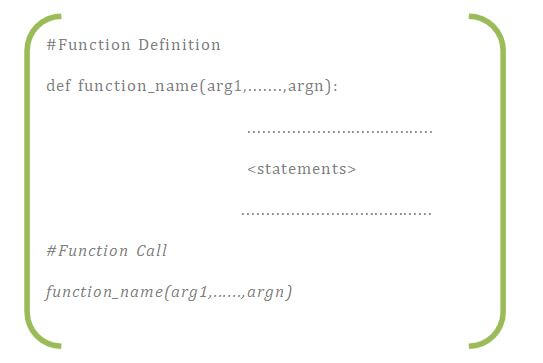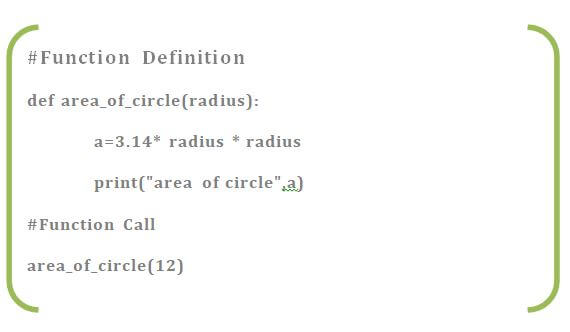#### with arguments and with return value

In this type,we pass arguments in caller function and value will pass in function definition.Here we are returning  value from function definition.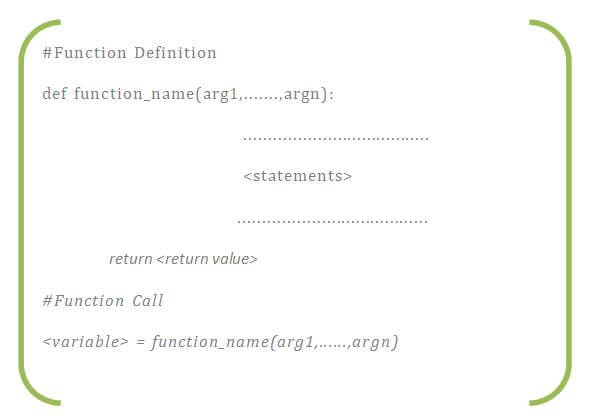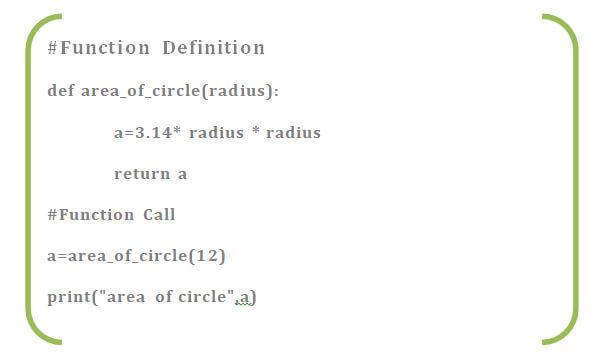#### without arguments and without return value:

In this type,we are not passing any  arguments in caller function to function definition.Here we are not returning any value from function definition.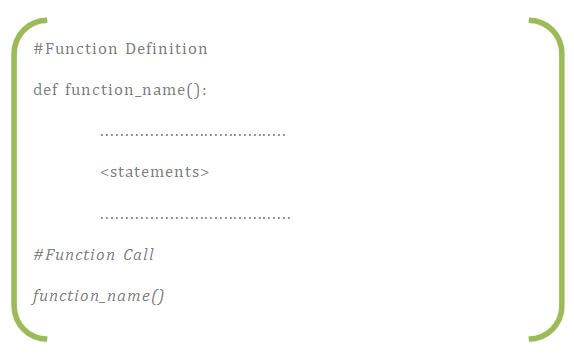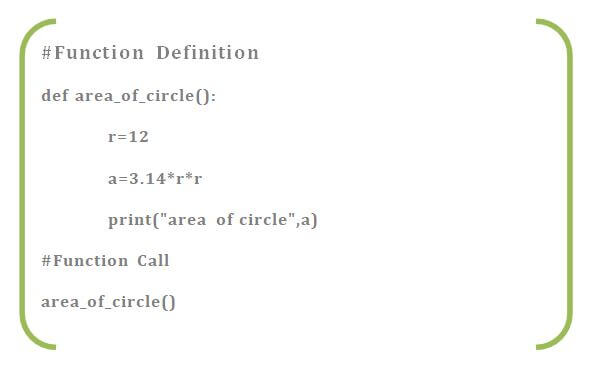#### without arguments and with return value:

In this type,we are not passing  arguments in caller function to  function definition.Here we are returning  value from function definition.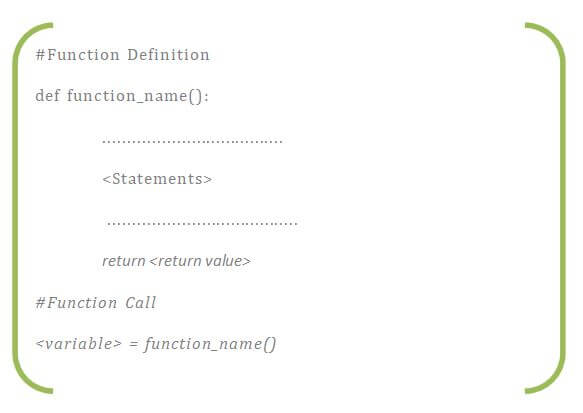#### Multiple return values from Function definition:

In this,we are returning more than one values from function definition.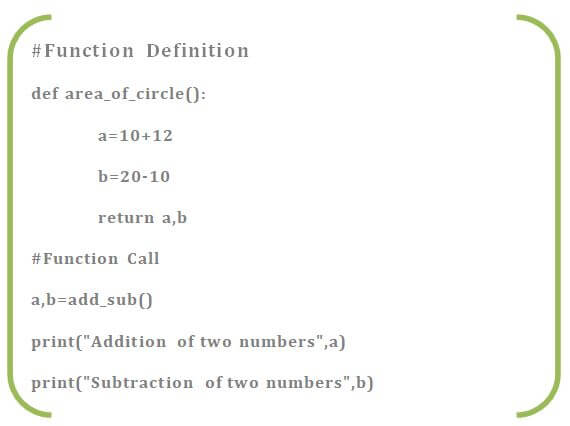### Python Docstring

A Python doctring can be used for documentation of a module, function, method or class. It is similar to a comment  and can be used to describe what a function does. Python docstrings are declared using triple double-quotations before and after adding the documentation as shown below. The docstrings can also be accessed by using __doc__. Note that there is a double underscore before and after “doc”.

 ```def greeting(name): """   This function greets to the person passed in as parameter         """ return "Hello, " + name + ". Good morning!" print(greeting.__doc__) print(greeting("Alice"))```

#### Output:

 ```This function greets to the person passed in as parameter Hello, Alice. Good morning!```

### Recursion in Python

Recursion is a function which calls itself. It can be set to call itself until it reaches a specific result. It is important to terminate the function upon meeting a specific condition so that it does not keep looping indefinitely. Below is an example in which recursion is used to find the factorial of a given integer.

 ```def checkFactorial(x): if x == 1: return 1 else: return (x * checkFactorial(x-1)) num = 4 print("The factorial of", num, "is", checkFactorial(num))```

#### Output:

 `The factorial of 4 is 24`

### Scope

The scope of a variable is the area of a program where the variable is recognized or accessible. In Python, there are two main types scopes of variables:

• Local variables
• Global variables

Variables and parameters that are defined within a function is only visible within the function in which it is declared and therefore, have a local scope. On the other hand, variables that are accessible from any part  or area of the program (including all the functions) have a global scope and are known as global variables.

### Pass by Object Reference Python

In Python, a variable is a reference to an object. A variable is not the object however. By assigning an object to the variable, the object can be accessed using the variable name. All the functions in Python are known to be called by object reference. This means that any changes made to the reference inside the function body is also reflected back outside the function where it was called. Hence, the values passed in the parameter can be modified within a function by calling it. In this example, num_list originally had only 3 values within the list. However, upon passing this list as reference within the function parameter, two more values have been appended within the function. The appended values along with the original values are accessible both in and out of the function.

 ```def updateList(num_list): num_list.append(40); num_list.append(50); print("list inside function = ",num_list) num_list = [10,20,30] updateList(num_list); print("list outside function = ",num_list);```

#### Output

 ```List inside function = [10, 20, 30, 40, 50] List outside function = [10, 20, 30 40, 50]```
However, in case of mutable objects, changes made to it would not reflect back to the original string. Rather, in this example,  two different string objects are made in which two different objects are printed.
 ```def updateSentence (sentence): sentence = sentence + " It’s nice to meet you"; print("string inside function :",sentence); sentence = "Hello." updateSentence(sentence) print("string outside function :",sentence); ```

#### Output

 ```string inside function = Hello. It’s nice to meet you string outside function = Hello.```